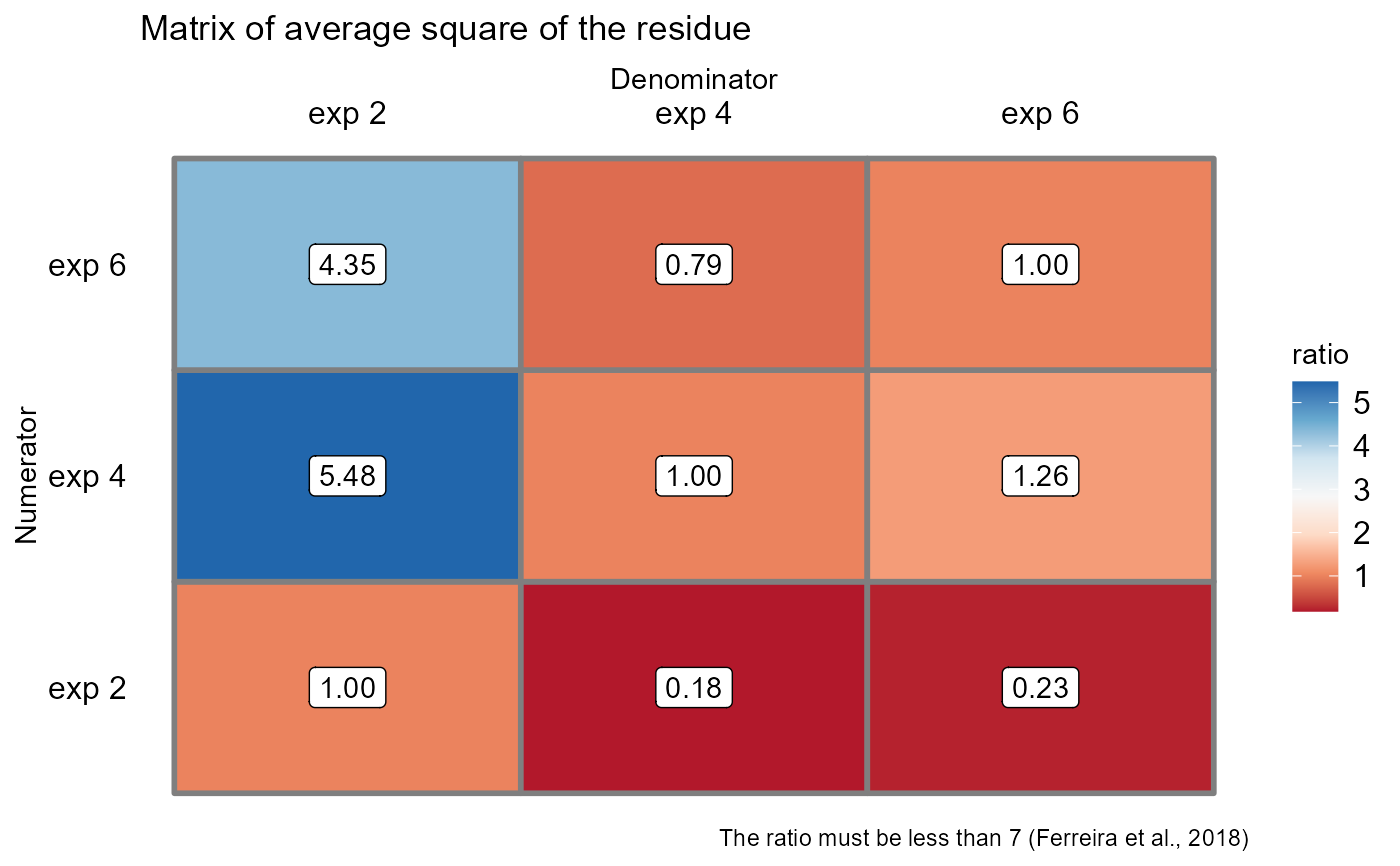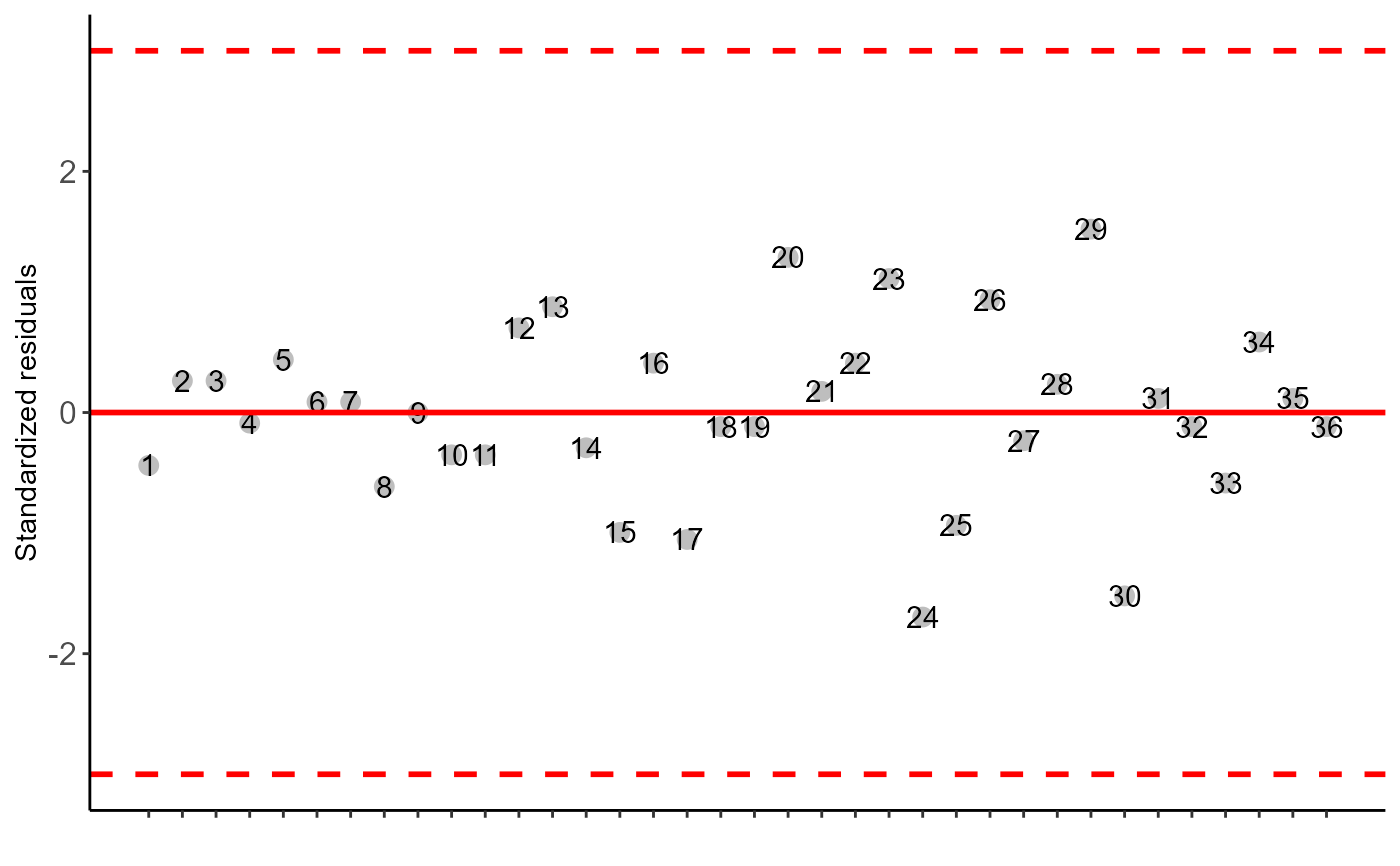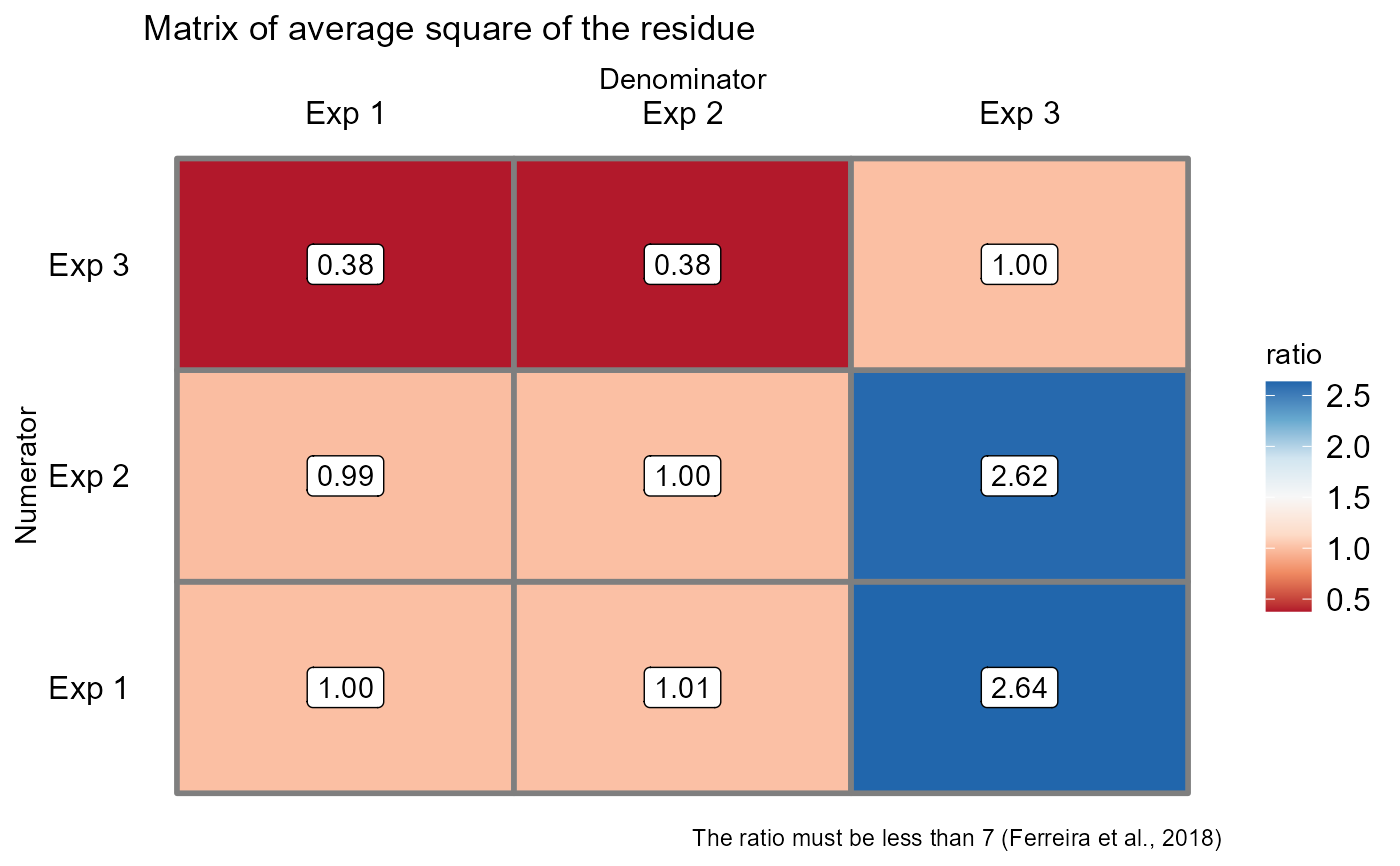Function of the AgroR package for joint analysis of experiments conducted in a randomized qualitative or quantitative single-block design with balanced data.

conjdbc(
trat,
block,
local,
response,
transf = 1,
norm = "sw",
homog = "bt",
theme = theme_classic(),
mcomp = "tukey",
quali = TRUE,
alpha.f = 0.05,
alpha.t = 0.05,
grau = NA,
ylab = "response",
title = "",
xlab = "",
fill = "lightblue",
angulo = 0,
textsize = 12,
dec = 3,
family = "sans",
errorbar = TRUE
)

## Arguments

trat

Numerical or complex vector with treatments

block

Numerical or complex vector with blocks

local

Numeric or complex vector with locations or times

response

Numerical vector containing the response of the experiment.

transf

Applies data transformation (default is 1; for log consider 0)

norm

Error normality test (default is Shapiro-Wilk)

homog

Homogeneity test of variances (default is Bartlett)

theme

ggplot2 theme (default is theme_classic())

mcomp

Multiple comparison test (Tukey (default), LSD, Scott-Knott and Duncan)

quali

Defines whether the factor is quantitative or qualitative (default is qualitative)

alpha.f

Level of significance of the F test (default is 0.05)

alpha.t

Significance level of the multiple comparison test (default is 0.05)

grau

Degree of polynomial in case of quantitative factor (default is 1)

ylab

Variable response name (Accepts the expression() function)

title

Graph title

xlab

Treatments name (Accepts the expression() function)

fill

Defines chart color (to generate different colors for different treatments, define fill = "trat")

angulo

x-axis scale text rotation

textsize

Font size

dec

Number of cells

family

Font family

errorbar

Plot the standard deviation bar on the graph (In the case of a segment and column graph) - default is TRUE

## Value

Returns the assumptions of the analysis of variance, the assumption of the joint analysis by means of a QMres ratio matrix, the analysis of variance, the multiple comparison test or regression.

## Note

The ordering of the graph is according to the sequence in which the factor levels are arranged in the data sheet. The bars of the column and segment graphs are standard deviation.

In the final output when transformation (transf argument) is different from 1, the columns resp and respo in the mean test are returned, indicating transformed and non-transformed mean, respectively.

## Author

Gabriel Danilo Shimizu, shimizu@uel.br

Leandro Simoes Azeredo Goncalves

Rodrigo Yudi Palhaci Marubayashi

## Examples

library(AgroR)
data(mirtilo)
with(mirtilo, conjdbc(trat, bloco, exp, resp))
#> Warning: Error() model is singular
#>
#> -----------------------------------------------------------------
#> Normality of errors
#> -----------------------------------------------------------------
#>                          Method Statistic   p.value
#>  Shapiro-Wilk normality test(W) 0.9812789 0.7876433
#>
#> As the calculated p-value is greater than the 5% significance level, hypothesis H0 is not rejected. Therefore, errors can be considered normal
#> -----------------------------------------------------------------
#> Homogeneity of Variances
#> -----------------------------------------------------------------
#>                               Method Statistic   p.value
#>  Bartlett test(Bartlett's K-squared)  1.094921 0.5784169
#>
#> As the calculated p-value is greater than the 5% significance level, hypothesis H0 is not rejected. Therefore, the variances can be considered homogeneous
#> -----------------------------------------------------------------
#> Independence from errors
#> -----------------------------------------------------------------
#>                  Method Statistic   p.value
#>  Durbin-Watson test(DW)  2.377661 0.4700462
#>
#> As the calculated p-value is greater than the 5% significance level, hypothesis H0 is not rejected. Therefore, errors can be considered independent
#> -----------------------------------------------------------------
#> Test Homogeneity of experiments
#> -----------------------------------------------------------------
#>  5.481481
#>
#> Based on the analysis of variance and homogeneity of experiments, it can be concluded that:
#> The experiments can be analyzed together#>
#>#>
#> -----------------------------------------------------------------
#> Analysis of variance
#> -----------------------------------------------------------------
#>                 Df     Sum Sq    Mean Sq    F value      Pr(>F)
#> Trat             2 14434.7222 7217.36111 45.9867257 0.001737072
#> Exp              2  4809.7222 2404.86111 15.3230088 0.013329484
#> Block/Local      6   362.5000   60.41667  0.3849558 0.857661006
#> Exp:Trat         4   627.7778  156.94444  0.7730901 0.556825561
#> Average residue 21  4263.1944  203.00926
#>
#> -----------------------------------------------------------------
#> Multiple comparison test ( tukey )
#> -----------------------------------------------------------------
#>        resp groups
#> 18 72.91667      a
#> 12 68.33333      a
#> 6  28.33333      b
#> []#>